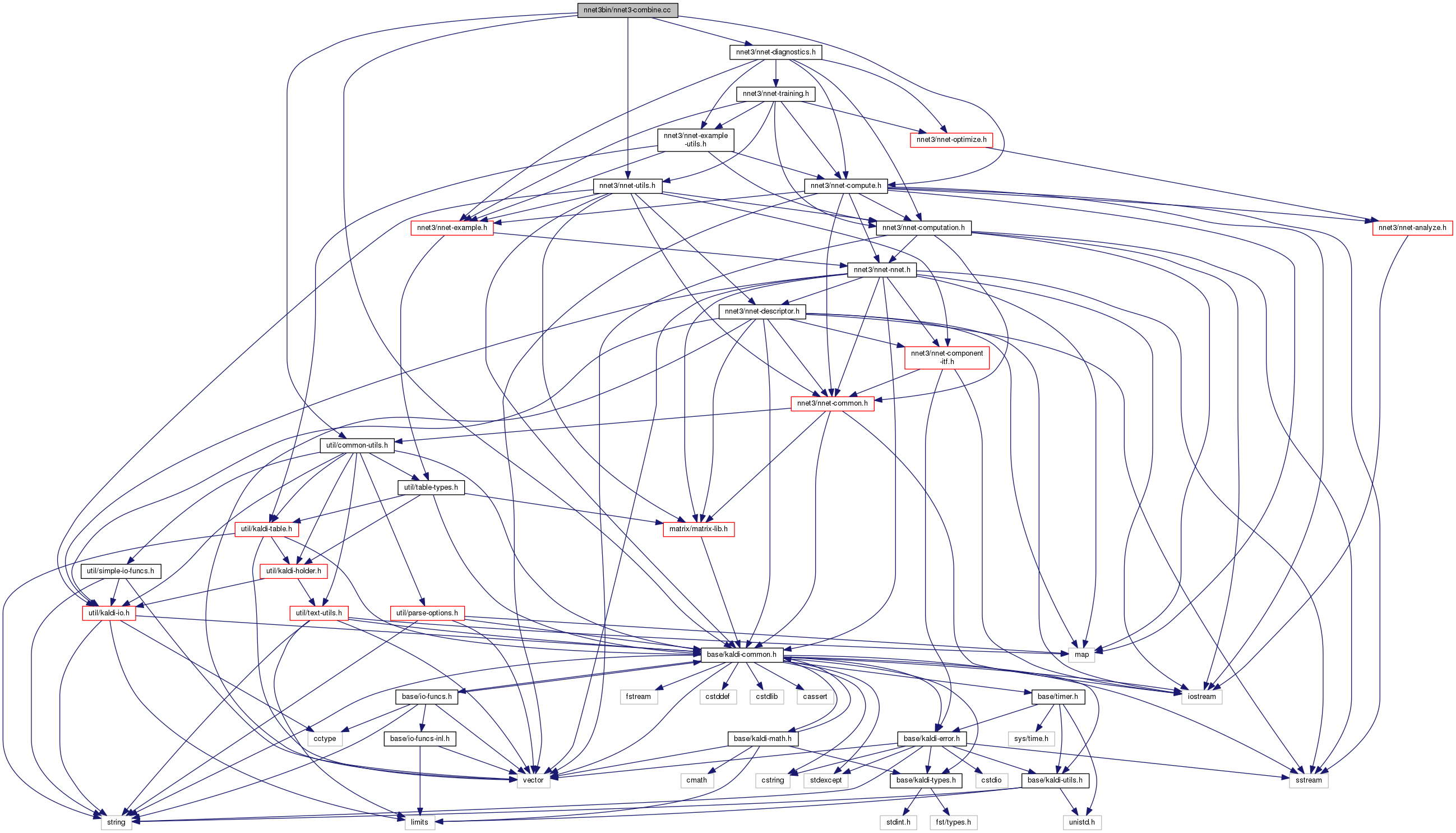nnet3-combine.cc File Reference
`#include "base/kaldi-common.h"`
`#include "util/common-utils.h"`
`#include "nnet3/nnet-utils.h"`
`#include "nnet3/nnet-compute.h"`
`#include "nnet3/nnet-diagnostics.h"`
Include dependency graph for nnet3-combine.cc:Go to the source code of this file.

## Namespaces

kaldi
This code computes Goodness of Pronunciation (GOP) and extracts phone-level pronunciation feature for mispronunciations detection tasks, the reference:

kaldi::nnet3

## Functions

double ComputeObjf (bool batchnorm_test_mode, bool dropout_test_mode, const std::vector< NnetExample > &egs, const Nnet &nnet, NnetComputeProb *prob_computer)

void UpdateNnetMovingAverage (int32 num_models, const Nnet &nnet, Nnet *moving_average_nnet)

int main (int argc, char *argv[])

## ◆ main()

 int main ( int argc, char * argv[] )

Definition at line 76 of file nnet3-combine.cc.

76  {
77  try {
78  using namespace kaldi;
79  using namespace kaldi::nnet3;
80  typedef kaldi::int32 int32;
81  typedef kaldi::int64 int64;
82
83  const char *usage =
84  "Using a subset of training or held-out examples, compute the average\n"
85  "over the first n nnet3 models where we maxize the objective function\n"
86  "for n. Note that the order of models has been reversed before\n"
87  "being fed into this binary. So we are actually combining last n models.\n"
88  "Inputs and outputs are 'raw' nnets.\n"
89  "\n"
90  "Usage: nnet3-combine [options] <nnet-in1> <nnet-in2> ... <nnet-inN> <valid-examples-in> <nnet-out>\n"
91  "\n"
92  "e.g.:\n"
93  " nnet3-combine 1.1.raw 1.2.raw 1.3.raw ark:valid.egs 2.raw\n";
94
95  bool binary_write = true;
96  int32 max_objective_evaluations = 30;
97  bool batchnorm_test_mode = false,
98  dropout_test_mode = true;
99  std::string use_gpu = "yes";
100
101  ParseOptions po(usage);
102  po.Register("binary", &binary_write, "Write output in binary mode");
103  po.Register("max-objective-evaluations", &max_objective_evaluations, "The "
104  "maximum number of objective evaluations in order to figure "
105  "out the best number of models to combine. It helps to speedup "
106  "if the number of models provided to this binary is quite "
107  "large (e.g. several hundred).");
108  po.Register("batchnorm-test-mode", &batchnorm_test_mode,
109  "If true, set test-mode to true on any BatchNormComponents "
110  "while evaluating objectives.");
111  po.Register("dropout-test-mode", &dropout_test_mode,
112  "If true, set test-mode to true on any DropoutComponents and "
113  "DropoutMaskComponents while evaluating objectives.");
114  po.Register("use-gpu", &use_gpu,
115  "yes|no|optional|wait, only has effect if compiled with CUDA");
116
118
119  if (po.NumArgs() < 3) {
120  po.PrintUsage();
121  exit(1);
122  }
123
124 #if HAVE_CUDA==1
125  CuDevice::Instantiate().SelectGpuId(use_gpu);
126 #endif
127
128  std::string
129  nnet_rxfilename = po.GetArg(1),
130  valid_examples_rspecifier = po.GetArg(po.NumArgs() - 1),
131  nnet_wxfilename = po.GetArg(po.NumArgs());
132
133  Nnet nnet;
135  Nnet moving_average_nnet(nnet), best_nnet(nnet);
136  NnetComputeProbOptions compute_prob_opts;
137  NnetComputeProb prob_computer(compute_prob_opts, moving_average_nnet);
138
139  std::vector<NnetExample> egs;
140  egs.reserve(10000); // reserve a lot of space to minimize the chance of
141  // reallocation.
142
143  { // This block adds training examples to "egs".
145  valid_examples_rspecifier);
148  KALDI_LOG << "Read " << egs.size() << " examples.";
149  KALDI_ASSERT(!egs.empty());
150  }
151
152  // first evaluates the objective using the last model.
153  int32 best_num_to_combine = 1;
154  double
155  init_objf = ComputeObjf(batchnorm_test_mode, dropout_test_mode,
156  egs, moving_average_nnet, &prob_computer),
157  best_objf = init_objf;
158  KALDI_LOG << "objective function using the last model is " << init_objf;
159
160  int32 num_nnets = po.NumArgs() - 2;
161  // then each time before we re-evaluate the objective function, we will add
162  // num_to_add models to the moving average.
163  int32 num_to_add = (num_nnets + max_objective_evaluations - 1) /
164  max_objective_evaluations;
165  for (int32 n = 1; n < num_nnets; n++) {
166  ReadKaldiObject(po.GetArg(1 + n), &nnet);
167  // updates the moving average
168  UpdateNnetMovingAverage(n + 1, nnet, &moving_average_nnet);
169  // evaluates the objective everytime after adding num_to_add model or
170  // all the models to the moving average.
171  if ((n - 1) % num_to_add == num_to_add - 1 || n == num_nnets - 1) {
172  double objf = ComputeObjf(batchnorm_test_mode, dropout_test_mode,
173  egs, moving_average_nnet, &prob_computer);
174  KALDI_LOG << "Combining last " << n + 1
175  << " models, objective function is " << objf;
176  if (objf > best_objf) {
177  best_objf = objf;
178  best_nnet = moving_average_nnet;
179  best_num_to_combine = n + 1;
180  }
181  }
182  }
183  KALDI_LOG << "Combining " << best_num_to_combine
184  << " nnets, objective function changed from " << init_objf
185  << " to " << best_objf;
186
187  if (HasBatchnorm(nnet))
188  RecomputeStats(egs, &best_nnet);
189
190 #if HAVE_CUDA==1
191  CuDevice::Instantiate().PrintProfile();
192 #endif
193
194  WriteKaldiObject(best_nnet, nnet_wxfilename, binary_write);
195  KALDI_LOG << "Finished combining neural nets, wrote model to "
196  << nnet_wxfilename;
197  } catch(const std::exception &e) {
198  std::cerr << e.what() << '\n';
199  return -1;
200  }
201 }
This code computes Goodness of Pronunciation (GOP) and extracts phone-level pronunciation feature for...
Definition: chain.dox:20
void UpdateNnetMovingAverage(int32 num_models, const Nnet &nnet, Nnet *moving_average_nnet)
kaldi::int32 int32
This class is for computing cross-entropy and accuracy values in a neural network, for diagnostics.
double ComputeObjf(bool batchnorm_test_mode, bool dropout_test_mode, const std::vector< NnetExample > &egs, const Nnet &nnet, NnetComputeProb *prob_computer)
void ReadKaldiObject(const std::string &filename, Matrix< float > *m)
Definition: kaldi-io.cc:832
The class ParseOptions is for parsing command-line options; see Parsing command-line options for more...
Definition: parse-options.h:36
void RecomputeStats(const std::vector< NnetChainExample > &egs, const chain::ChainTrainingOptions &chain_config_in, const fst::StdVectorFst &den_fst, Nnet *nnet)
This function zeros the stored component-level stats in the nnet using ZeroComponentStats(), then recomputes them with the supplied egs.
struct rnnlm::@11::@12 n
A templated class for reading objects sequentially from an archive or script file; see The Table conc...
Definition: kaldi-table.h:287
bool HasBatchnorm(const Nnet &nnet)
Returns true if nnet has at least one component of type BatchNormComponent.
Definition: nnet-utils.cc:527
#define KALDI_ASSERT(cond)
Definition: kaldi-error.h:185
void WriteKaldiObject(const C &c, const std::string &filename, bool binary)
Definition: kaldi-io.h:257
#define KALDI_LOG
Definition: kaldi-error.h:153